Digital Clock Replacement Board for E21

Wichit Sirichote, wichit.sirichote@gmail.com

Build a 12/24 digital clock for BMW E21 dash board with the 89S52 microcontroller.

Available now replacement kit.

This page describes how to build the microcontroller circuit for replacing the E21 digital clock. The obsolete SCL5455E automotive clock integrate circuit was replaced by the ATMEL 89S52 8-bit microcontroller running with a 12MHz oscillator. The firmware is timer0 interrupt that produces 10ms tick. This tick is used to produce 12/24 clock. The board was modified using wire soldering. The replacement board can be plugged back to the power terminals provided by the tachometer board directly.Figure 1: The replacement board using the 89S52 microcontroller.

The original clock board built with the SCL5455E chip is shown in Figure 2.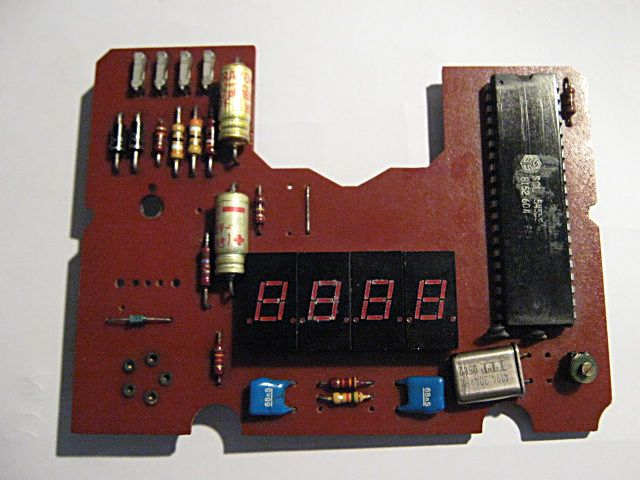Figure 2: The original PCB using the SCL5455E.Figure 3: The bottom copper layer.

Hardware Schematic

The microcontroller, U2 is the 8-bit 89S52 microcontroller. The chip can be programmed using Asim loader through the ISP port easily. The display has 4-digit, using the HP5082-7331 common anode 7-segment LED. The 7-segment LED is driven with sinking current through the microcontroller parallel port, P0, P1, P2 and P3. The power source for driving such LED is supplied from +3.3V voltage regulator through ignition power terminal (R). U2 is powered by D4, +3.3V zener diode through +12V battery terminal. When ignition switch is turned off, , the LED display will be turned off, but the microcontroller instead is still functioning by a +12V battery power. The power drawn by D4 and microcontroller is about 20mA @+12V or 240mW. When the display on, total power will be 1.2W. J1 is soldering pad used for selecting between 12 or 24 clock.

Figure 4: Hardware schematic.

HP 5082-7731 seven segment LED

The part number for 7-segment LED is HP 5082-7731 common anode LED.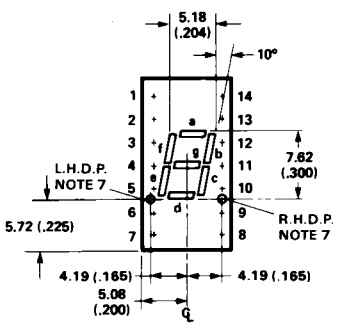Figure 5: Dimension of HP5082-7331 LED (inches).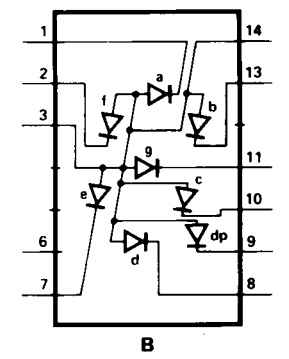Figure 6: Pin designation for each segments.

Rebuild the Board

The original board was rebuilt by removing copper traces at the 40-pin pads, 7-segment pads and power pads. Circuit connection is done by wire soldering.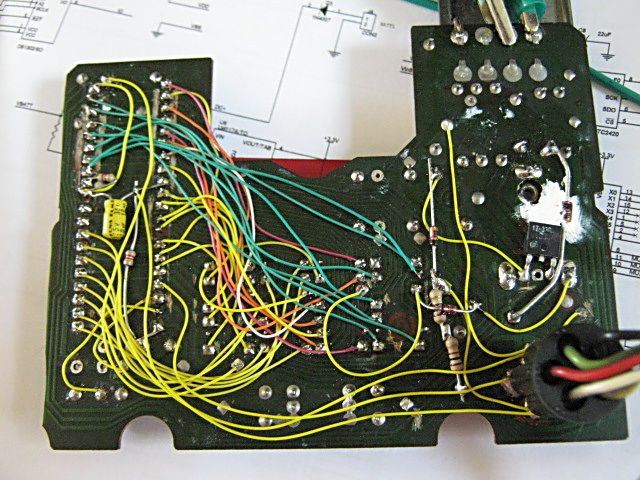Figure 7: Wire soldering and copper traces removing.

` `
 `Bill Of Materials May 23,2010 11:41:26 Page1` ```Item Quantity Reference Part ______________________________________________``` ```1 2 C1,C3 30pF 2 1 C2 10uF 3 2 C4,C5 47uF 16V 4 1 C6 0.1uF 5 4 D1,D2,D5,D6 1N4001 6 1 D4 1N5226 7 1 JP1 CONN AV 5-P 8 4 JR1,JR2,JR3,JR4 CONN RECT 1 9 1 J1 CON2 10 1 Q1 12MHz Xtal 11 1 R1 4.7k 12 1 R2 10k 13 1 R3 1k 0.5W 14 4 U1,U3,U4,U5 HP5082-7731 15 1 U2 AT89S52 16 1 U6 LM117A/+3.3V```
```
Software
```

The firmware produces 10ms tick by using timer0 interrupt. The realtime clock is updated every 10ms. The display is updated every second and when h or min button are pressed.
 ```/* Replacement for E21 clock SCL5455E chip The 89S52 microcontroller running at 12Mhz uses Timer0 to produce 10ms tick. Check xtal oscillation frequency by measuring the ALE pin. The ALE clock is 1/6 of Xtal frequency. Fine adjusment may be done by TL0 reload value. TIME key adjust time within +/-2mins at a given hour to the correct clock. Hour and Min keys have repeat function. Copyright (C) 2010 W.Sirichote, wichit.sirichote@gmail.com This program is free software: you can redistribute it and/or modify it under the terms of the GNU General Public License as published by the Free Software Foundation, either version 3 of the License, or (at your option) any later version. This program is distributed in the hope that it will be useful, but WITHOUT ANY WARRANTY; without even the implied warranty of MERCHANTABILITY or FITNESS FOR A PARTICULAR PURPOSE. See the GNU General Public License for more details. You should have received a copy of the GNU General Public License along with this program. If not, see . */ ``` ```#include /* special function register declarations */ #include ``` ```sfr WDTRST = 0xa6; sbit key_hour = P2^7; sbit key_min = P3^7; sbit H24_12 = P0^7; // select between 24 or 12 clock, open for 24, Ground for 12``` ```unsigned char sec100,sec,min,hour,flag1; unsigned char hour_max;``` ```unsigned char i,digit, buffer; char cputick,key,delay,count1; char press_hour=0; char press_min=0; unsigned char convert = {0x3F,0x06,0x5b,0x4f,0x66,0x6d,0x7d,0x07,0x7f,0x6f};``` ```char timer4=0; char timer5=0; char timer6=0;``` ```void time(); void time2(); void timeToBuffer();``` `void write_digit();` ```void set_hour(); void set_min(); void scan_key(); void release_key();``` ```void auto_repeat(); void clear_wdt(); ``` ```static timer0int (void) interrupt 1 using 1 { TH0 |= 0xd8; // reload timer 0 with d8f0h //TL0 |= 0xf0; // actual calculation is 0xf0 TL0 |= 0xff; // adjustment cputick++; time(); // update realtime clock // P0^=0x80; // toggle P0.7 for 100 Hz timer base (100/2=50Hz) } ``` ```void long_delay(unsigned int j) { unsigned int i; for(i=0; i23 || hour <0 || min>59 || min <0) { hour=0; min=0; sec=0; } }``` `// adjust time within +/-2mins` ```void time_tone() { if(min>=58) { min=0; sec=0; sec100=0; // increment hour by one if(H24_12==1) hour_max=24; else hour_max=13;``` ``` if (++hour >= hour_max) { if(H24_12==0) hour = 1; else hour = 0; } }``` ``` if(min<=2) { min=0; sec=0; sec100=0; } } ``` ```void main() { long_delay(100); TMOD = 0x01; PT0=0; ET0=1; TR0 = 1; EA=1; cputick =0; sec100=0; check_cold_boot(); // if cold boot and non clock value, reset them to 0:00, else use current value``` ``` time_tone(); ``` ``` flag1 = 0; count1 = 0; buffer = ~0x06; // display E21 when reset buffer = ~0x5b; buffer = ~0x79; buffer = ~0x00; write_digit();``` ``` while(1) { while (cputick==0) clear_wdt();``` ` cputick = 0;` `/*------------- the following tasks execute every 10ms ------*/` ``` timeToBuffer(); write_digit(); set_hour(); set_min(); auto_repeat(); release_key(); /*-----------------------------------------------------------*/ } }``` ```/* update real-time clock */ void time ()``` ```{ if (++sec100 >= 100) // 100 * 10 ms = 1 s tested with 10*10 = 100ms {sec100 = 0; flag1 |= 0x05; /* set bit 0, bit 2 */ if (++sec >= 60) {sec = 0; flag1 |= 0x02; /* set bit 1 */ if (++min >= 60) {min = 0;``` ``` H24_12=1; // make it to be input port if(H24_12==0) hour_max=13; else hour_max=24;``` ``` if (++hour >= hour_max) { if(H24_12==0)hour = 1; else hour = 0;``` ``` } } } } } ``` ```void write_digit() { P3=buffer; P2=buffer; P1=buffer; P0=buffer; }``` ```void timeToBuffer() { if(flag1&1) // run only when any key was pressed { flag1&=~1; buffer = ~convert[min%10]; // test buffer = ~convert[min/10]; buffer = ~convert[hour%10]; buffer = ~convert[hour/10]; buffer &= ~0x80; // make dot point if(buffer==~0x3F) buffer=0xff; } }``` ` ` ```void update_when_press() { buffer = ~convert[min%10]; // test buffer = ~convert[min/10]; buffer = ~convert[hour%10]; buffer = ~convert[hour/10]; buffer &= ~0x80; // make dot point if(buffer==~0x3F) buffer=0xff; P3=buffer; P2=buffer; P1=buffer; P0=buffer; }``` ` ` ```void set_hour() { if(key_hour==0 && press_hour==0) { press_hour=1; H24_12=1; if(H24_12==1) hour_max=24; else hour_max=13;``` ``` if (++hour >= hour_max) { if(H24_12==0) hour = 1; else hour = 0; } update_when_press(); }``` ``` ``` `}` ```void set_min() { if(key_min==0 && press_min==0) { press_min=1; if (++min >= 60) min = 0; sec=0; update_when_press(); } ``` `}` ```void release_key() { if(key_hour==1 && press_hour==1) { long_delay(1000); press_hour=0; } // debounce when released if(key_min==1 && press_min==1) { long_delay(1000); press_min=0; }``` `}` ```void auto_repeat() { if(key_min==0 && press_min==1) { if(++timer6>50) { timer6=0; press_min=0; } }``` ``` if(key_hour==0 && press_hour==1) { if(++timer6>50) { timer6=0; press_hour=0; } }``` ```} ```

Figure 8: Source code listing.

Clock settings and Time Tone key

The E21's dashboard provides three buttons for clock settings, i.e. TIME, h and min. TIME tone button shown in the center is used to reset the microcontroller and adjust clock within +/-2mins for a given hour.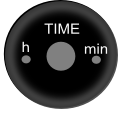Figure 9: Time settings button.

When key TIME tone button was pressed, the min and sec will be cleared to 00. The correcting zone is -2 to +2 minutes. For example if current minute is 58 mins, the hour will be incremented by one. But if it is 02 mins, the hour will not change. This feature is the same as the original board. The h and min buttons also have repeat function when press more than 0.5s.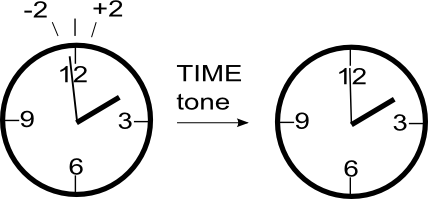Figure 10: Time tone pressing.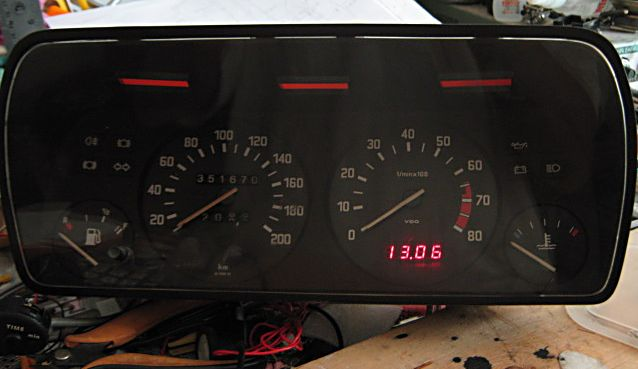Figure 11: Reinstall in the E21 instrument console, powered with +12V Lab power supply.

 Schematic E21clock.pdf C source code N/A Orcad Schematic File N/A Intel-Hex file E21clock.hex

BMW E21 Digital Clock Replacement kitAvailable now on eBay for 24 or 12 clock format.

Free optional toggle switch for time setting

Classic look display, brightness is adjustable.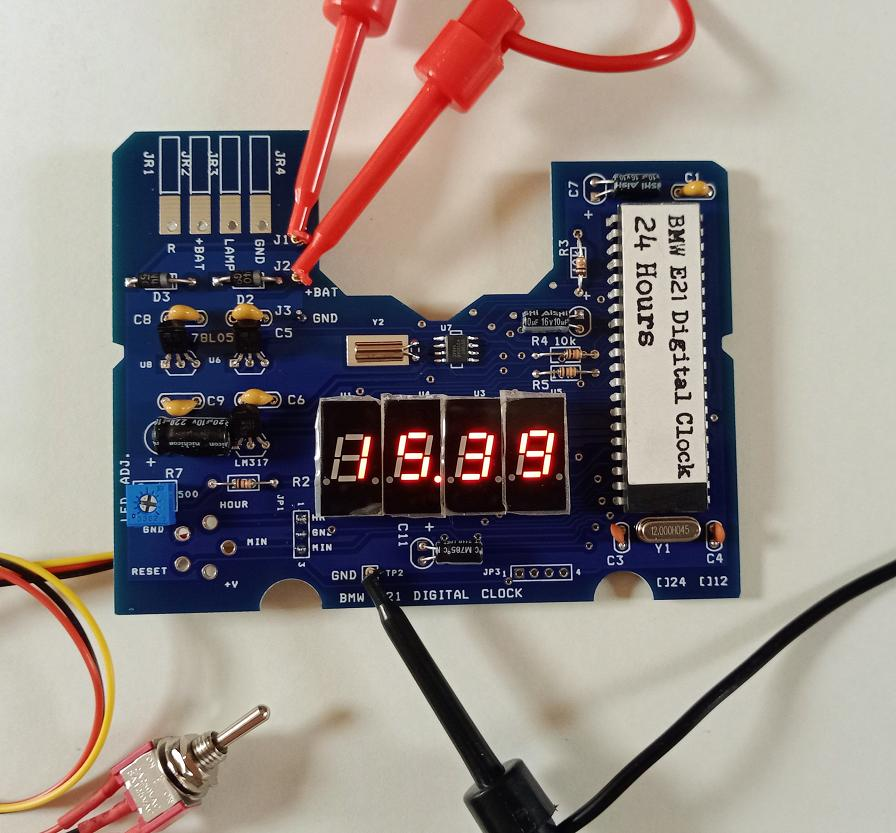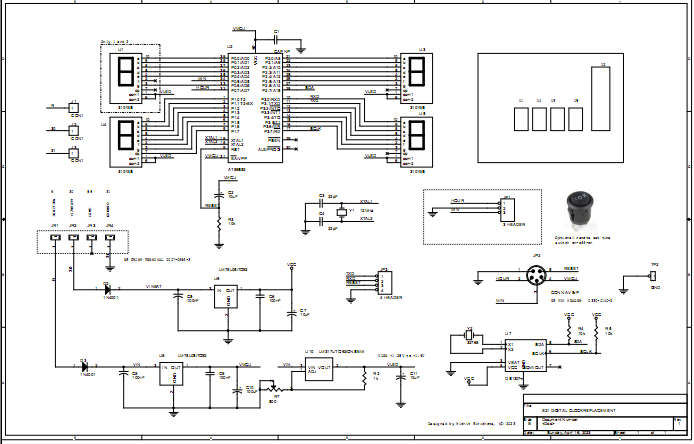Complete schematic, click to enlarge